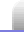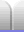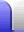A quadratic polynomial or quadratic expression is an expression which can be written as ax2 + bx + c, where a, b and c are constants.

A quadratic equation has an equals sign and can be written as ax2 + bx + c = 0.

Remember that the constants a and b in front of the x terms are called coefficients. The number c is simply a constant.

### Why factorise

Factorise and solve are key words in exam questions that tell students what they are expected to do to answer a question.

When we are dealing with a quadratic equation, having a 0 on one side is important, as it (often) allows us to solve the equation. If we can factorise, we know that the quadratic on one side of the equation can only equal zero if one of its factors equals zero. This means we can make an equation with each factor equal to zero, then solve for x.

x2 + 5x + 6 = 0
⇒ (x + 2)(x + 3) = 0
factorised

x + 2 = 0
or x + 3 = 0

x = -2
or x = -3
solved

### Special situations

Being able to recognise some special situations will save considerable time when factorising. It's good to be able to spot these before going to the trouble of labourious factorising.

#### Difference of two squares.

The name of this particular form comes from the way the middle term in the quadratic is missing (it has a zero coefficient) and the remaining two terms are both square numbers, with one substracted from the other.

It is factorised by taking the square root of each of the original terms, and using them in two factors with opposite signs.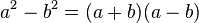Example:

4x2 – 9
= (2x + 3)(2x – 3)

Example featuring the difference of two quartics (the sum of two squares cannot be factorised with real factors):

x4 – y4
= (x2 + y2)(x2 – y2)
= (x2 + y2)(x + y)(x – y)

#### Perfect square.

This is when the quadratic equation has a single root that is repeated (and thus squared; it's multiplied by itself). The quadratic equation has a particular form where the first and last terms are both positive square numbers and the coefficient of the middle term is ±2 times the square root of the product of the first and last terms.

It is factorised by taking the square root of each of the first and last terms in the quadratic, and using them in a single factor which is then squared. The resulting factor is the square root of the quadratic palynomial.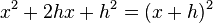There is a method known as completing the square which depends on this equation in order to be able to solve for x. The quadratic formula (below) is derived using this.

#### Sum and difference of cubics.

The sum and difference of two cubes can both be factorised. These are special cases which may be useful for senior students to know. Note which way the signs work out; the sign in the first factor (the simpler factor) is the same as the sign in the original sum or difference. The factorisation of the sum of two squares has a negative sign only for the xy term, while the difference of two cubes has a negative sign only in the first factor.

x3 + y3 = (x + y)(x2 – xy + y2)

x3 – y3 = (x – y)(x2 + xy + y2)

The second factors look tantalisingly close to the factorisation of a perfect square (see above), but the xy term here is missing the necessary coefficient of 2.

Example with a common factor in the coefficient:

16x3 + 54y3
= 2(8x3 + 27y3)
= 2(23x3 + 33y3)
= 2((2x)3 + (3y)3)
= 2(2x + 3y)((2x)2 – 2x3y + (3y)2)
= 2(2x + 3y)(4x2 – 6xy + 9y2)

Example mixing squares and cubics (the last line is just aesthetic tidying up):

y6 – x6
= (y3 + x3)(y3 – x3)
= (x + y)(x2 – xy + y2)(y – x)(x2 + xy + y2)
= (x2 + xy + y2)(x2 – xy + y2)(x + y)(y – x)

How do we do the factorising? Let's have a look at an example.

-18 – 3x2 = 15x

1. Make sure all the terms are on the same side and equal zero.

-18 – 3x215x = 0

2. Arrange all the terms so they are in descending order of power. (The x2 term first, then the x term, then the constant.)

-3x2 – 15x – 18 = 0

3. Make sure the x2 term is positive. If it's not change the sign of every term. (This is multiplying by -1 and is the same as taking everything to the other side. If you are dealing with an inequality this changes the direction of the inequality.)

3x2 + 15x + 18 = 0

4. Simplify the quadratic equation if possible. Check the three terms for common factors. For example, are all the coefficients even?

x2 + 5x + 6 = 0

5.  If the x2 coefficient is a prime number we can write out the brackets with ax and x in the two sets of brackets, where a is the prime number. 3x2 + 10x + 8 = 0 (3x      )(x      ) = 0
If the x2 coefficient is 1 (so the first term is just x2) we can simply write out the brackets with an x in each set.

x2 + 5x + 6 = 0
(x      )(x      ) = 0

Don't forget to write the = 0; it's still an equation.

6. In the quadratic, if the sign of the constant is positive, the two signs in the factors are the same. (Two positives multiplied together make a positive, two negatives multiplied together make a positive.) The sign of the x term is the same as the signs in the two factors.

OR if the sign of the constant is negative, the two signs in the factors are different.

x2 + 5x + 6 = 0
(x +   )(x +   ) = 0

x2 5x + 6 = 0
(x   )(x   ) = 0

x2 + 5x 6 = 0
(x +   )(x   ) = 0

7.  3x2 + 10x + 8 = 0 1, 8 2, 4
We now look for two numbers that multiply to make the constant. Make a list of the factor pairs of the constant. (Remember that you only need to go up to the square root with the first of the pair, and there are factorising tricks to help.)

 x2 + 5x + 6 = 0 1, 6 2, 3
8.  If the x2 coefficient is not 1 then remember it will multiply by the number in the other factor when finding the sum. 3x2 + 10x + 8 = 0 (3x      )(x      ) = 0 4 + 3*2 = 10 (3x + 4)(x + 2) = 0
If the signs in the two factors are the same we are looking for a factor pair with a sum that equals the x coefficient.

OR if the signs are different we are looking for a factor pair with a difference that equals the x coefficient. The larger number of the factor pair goes in the factor with the same sign as the x term.

 x2 + 5x + 6 = 0 (x +   )(x +   ) = 0 2 + 3 = 5 (x + 2)(x + 3) = 0 x2 – 5x + 6 = 0 (x –   )(x –   ) = 0 2 + 3 = 5 (x – 2)(x – 3) = 0 x2 + 5x - 6 = 0 (x +   )(x –   ) = 0 6 – 1 = 5 (x + 6)(x – 1) = 0

If the quadratic is even more complicated there is a more elaborate approach that might work. Lets look at a couple of examples.

 8x2 + 29x – 12 = 0 9x2 – 85x + 36 = 0
1. Check for common factors in case the equations can be simplified.

2. The signs in the two factors can be determined as in the previous instructions.

3.  8x2 + 29x - 12 = 0 (    +   )(    –   ) = 0 9x2 – 85x + 36 = 0 (    –   )(    –   ) = 0
4. Neither the x2 coefficient (a) nor the constant (c) is prime, so there are multiple different options for how they could be factorised. Multiply a by c and make a list of the product's factor pairs. When making this list remember you don't need to consider any factors which are not already factors of a or c, so that can save some time checking factors.

5.  8x2 + 29x – 12 = 0 8 * 12 = 96 1, 96 2, 48 3, 32 4, 24 6, 16 8, 12 9x2 – 85x + 36 = 0 9 * 36 = 3 * 108 = 324 1, 324 2, 162 3, 108 4, 81 6, 54 9, 36 12, 27 18 (this is √324)
6. If the signs in the two quadratic factors are the same, find a factor pair in the list which has a sum the same as the x coefficient.
OR if the signs in the two factors are different, find a factor pair in the list which has a difference the same as the x coefficient.

The x coefficient is split into two parts with this factor pair as their coefficients. (It doesn't matter which part is listed first.)

7.  8x2 + 29x – 12 = 0 32 – 3 = 29 8x2 – 3x + 32x – 12 = 0 9x2 – 85x + 36 = 0 4 + 81 = 85 9x2 – 81x – 4x + 36 = 0
8. Take out common factors from each pair of terms. What's left in brackets should be the same, so combine the factors outside the brackets into one factor.

9.  8x2 – 3x + 32x – 12 = 0 x(8x – 3) + 4(8x – 3) = 0 (x + 4)(8x – 3) = 0 8x2 + 32x – 3x – 12 = 0 8x(x + 4) – 3(x + 4) = 0 (8x – 3)(x + 4) = 0 9x2 – 81x – 4x + 36 = 0 9x(x – 9) – 4(x – 9) = 0 (9x – 4)(x – 9) = 0 9x2 – 4x – 81x + 36 = 0 x(9x – 4) – 9(9x – 4) = 0 (x – 9)(9x – 4) = 0

If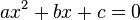thenIf factorising a quadratic equation is too tricky we can use the quadratic formula to solve it. Students should remember this formula.

The part inside the square root sign is called the discriminant.

• If the discriminant is positive, x will have two different values. (Also called "two real roots", which if the quadratic equation is graphed are the x values at which the parabola intercepts the x axis.)

• If the discriminant is zero, x will have only one value. (Also called "one real root" or "a repeated root", where on a graph the parabola just touches the x axis.) This means the quadratic equation can be factorised as a perfect square.

• If the discriminant is negative, x will have no real values. (Also called "no real roots", although in year 13 maths, complex numbers are introduced which can deal with this.)

The quadratic formula is derived by completing the square (see perfect square in Special situations above). This can be done a number of ways, such as starting by dividing each term by a, but a simpler way is to start by multiplying each term by 4a. This is because √4 = 4/2 (or 2*2 = 2+2), which makes it easy to complete the square. Each step of this method puts one more term of the finished formula in place.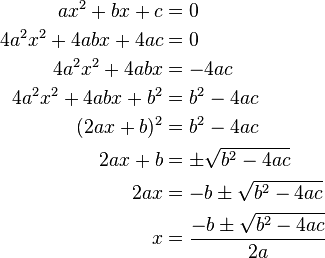A quadratic is a 2nd order polynomial, which means the greatest exponent is 2. There are also formulae for solving 3rd and 4th order polynomials, but they are much more complicated than the quadratic formula. After that it gets much more complicated again, which is why even really good scientific calculators stop with solving 4th order polynomials. See this page for more information.Home Astronomy Chemistry Electronics & Computers Mathematics Physics Field Trips Turn on javascript for email link